0
4311

# Logical Reasoning Questions For SBI PO PDF

Download SBI PO Logical Reasoning Questions & Answers PDF for SBI Clerk Prelims and Mains exam. Very Important SBI PO Logical Reasoning questions on with solutions.

Take a Free SBI PO Mock Test

Question 1: Select the related word/letters/number from the given alternatives.
81 : 10 :: 169 : ?

a) 23

b) 18

c) 12

d) 14

Question 2: If “!” denotes “added to”, “@” denotes “divided by”, “%” denotes “multiplied by” and “^” denotes “subtracted from”, then 13 ! 102 @ 6 % 2 ^ 41 = ?

a) 6

b) 9

c) 14

d) 12

Question 3: Pointing to the photograph, Ram said: “She is the only daughter of my father’s mother”. How is Ram related to the person in the photograph?

a) aunt

b) son

c) nephew

d) grandson

Question 4: If 1st January 1897 fell on a Friday, on what day of a week 1st January of 1901 would have fallen on?

a) Sunday

b) Thursday

c) Wednesday

d) Tuesday

Question 5: If 121 @ 34 = 87 and 28 ! 13 = 41 then what is the value of
353 ! 89 @ 167?

a) 275

b) 263

c) 317

d) 244

Question 6: A machine codes EAT as 100 and CAT as 60. Which of the following words will be coded as 40320?

a) BAIN

b) PAINT

c) HIGH

d) RAISE

Question 7: Find the order in which the following words will appear in a dictionary.
2.Quark
4.Quartet

a) 2143

b) 3124

c) 3214

d) 1324

Question 8: Virat first travels some distance in the west direction, then he takes a right and walks for some time. After that, he again takes a right and travels for some time. Finally, he takes a left and reaches his destination in some time. In which direction did he proceed just before reaching the destination?

a) North

b) South

c) East

d) West

Question 9: In this question, the sets of numbers given in the alternatives are represented. The columns and rows of Matrix I are numbered from 0 to 4 and that of Matrix II are numbered from 5 to 9. A letter from these matrices can be represented first by its row and next by its column, e.g., ‘V’ can be represented by 57, 79, etc., and ‘G’ can be represented by 76, 21, etc. Similarly, you have to identify the set for the word “SWINDLE”.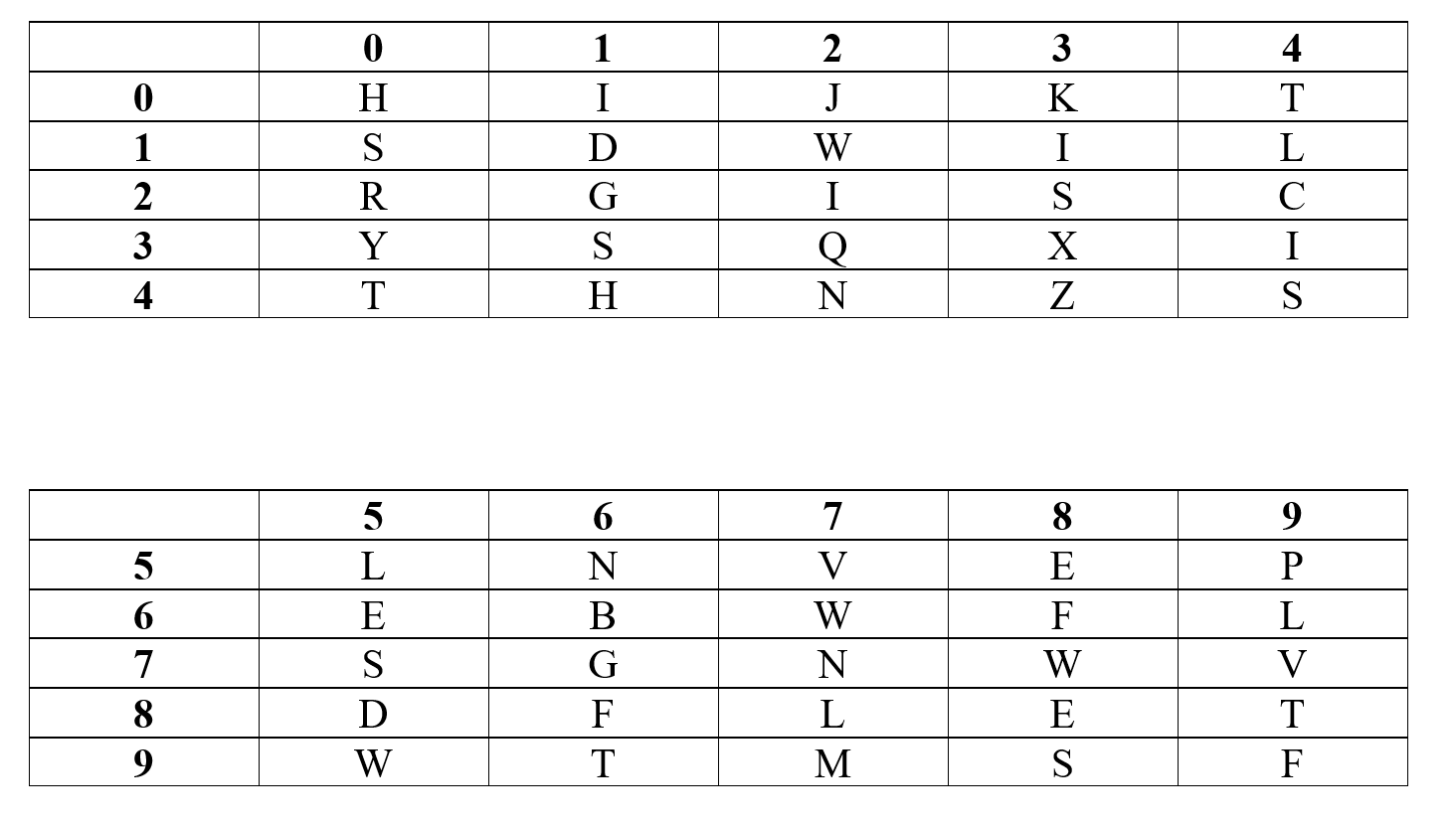a) 75, 67, 13, 57, 85, 87, 88

b) 31, 12, 34, 77, 11, 86, 58

c) 23, 67, 76, 56, 85, 86, 65

d) 23, 95, 34, 77, 11, 87, 65

Question 10: From the given alternative words, select the word which can be formed using the letters of the given word?
GSPLQTIEQNU

a) STURDY

b) STERN

c) LISTEN

d) STEM

Question 11: In the following question, select the missing number form the given series.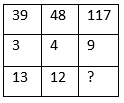a) 9

b) 7

c) 8

d) 13

Question 12: A series is given, with one term missing. Choose the correct alternative from the given ones that will complete the series.
-3, -1, 11, 39, 89, 167, ?

a) 277

b) 278

c) 279

d) 281

Question 13: Select the odd one out from the given alternatives.

a) 57

b) 51

c) 29

d) 91

Question 14: Five friends, Deepak, Rahul, Mukesh, Ajit and Sohan are standing in a queue in order of decreasing weight. Deepak is standing between Mukesh and Ajit and they all are standing consecutively. Mukesh is heavier than Ajit and Sohan. Deepak is lighter than Rahul.
Who is the heaviest?

a) Mukesh

b) Rahul

c) Deepak

d) Either Mukesh or Rahul

Question 15: Some children are standing in a row. Ram is fourth from the left while Rahul is third from the right. If Ram and Rahul interchange their position then Rahul is sixth from the right. What will be the position of Ram from the left after the interchange?

a) 8th

b) 6th

c) 9th

d) 7th

Question 16: Consider the given statement/s to be true and decide which of the given conclusions/assumptions can definitely be drawn from the given statement.
Statements:
A : Mid-day meal program has managed to increase the attendance of students.
B: But, there is a sharp decline in the number of students in the afternoon session.
Conclusion:
I. Mid-day meal program has rekindled the interest to study among the students.
II. Some students come to school just to avail a free meal.

a) Choose A if only conclusion I follows.

b) Choose B if only conclusion II follows.

c) Choose C if both conclusion I and II follow.

d) Choose D if neither conclusion I nor conclusion II follows.

Question 17: In each of the following questions two statements are given, and these statements are followed by two conclusions numbered (1) and (2). You have to take the given two statements to be true even if they seem to be at variance from commonly known facts. Read the conclusions and then decide which of the given conclusions logically follows from the two given statements, disregarding commonly known facts.
Statements :
Some fishes are alligators. All the alligators are cats.Conclusions :
(1) Some fishes are cats.
(2) No alligator is fish.

a) Only (1) conclusion follows

b) Only (2) conclusion follows

c) Either (1) or (2) follows

d) Neither (1) nor (2) follows

Question 18: According to the following venn diagram how many teachers do not read books?

a) 69

b) 66

c) 79

d) 74

Question 19: Which of the following correctly depicts the mirror image of ‘INSTINCTIVE’?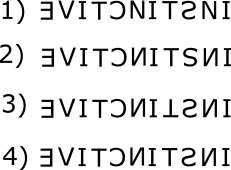a) 1

b) 2

c) 3

d) 4

Question 20: Select the word which CANNOT be formed using below word
CONVERSATION

a) VERSION

b) CONSERVATION

c) NATION

d) STATION

$\sqrt{81} + 1 = 10$
$\sqrt{169} + 1 = 14$
Hence, option D is the correct option.

13 ! 102 @ 6 % 2 ^ 41 = 13 + [(102 /6) * 2] – 41 = 13 + (17*2) – 41 = 6
Hence, option A is the right choice.

The person in the photograph should be Ram’s aunt. Thus, Ram is her nephew.

1st January of consecutive non -leap years will fall on consecutive days since 365 leaves a remainder of 1 on division by 7.

1900 is not a leap year. 1900 is divisible by 100 but not by 400. Therefore, all the years from 1897 till 1901 are non-leap years.

Therefore, the day on which 1st January falls will move by 1 for every year. Therefore, in 1897, 1st January would have fallen on Saturday. In 1898, 1st January would have fallen on Sunday. Similarly, in 1901, 1st January would have fallen on Tuesday. Therefore, option D is the right answer.

121 @ 34 = 87 and 28 ! 13 = 41
121 – 34 = 87 and 28 + 13 = 41
So, 353 ! 89 @ 167 = 353 + 89 – 167 = 275
Hence, option A is right choice.

The numerical position of E is 5, A is 1 and T is 20 – 5*1*20 = 100.
The numerical position of C is 3, A is 1 and T is 20 – 3*1*20 = 60.

BAIN = 2*1*9*14 = 252.
PAINT = 16*1*9*14*20 = 40320.
HIGH = 8*9*7*8 = 4032.
RAISE = 18*1*9*19*5 = 15390.
As we can see, PAINT will be coded as 40320. Therefore, option B is the right answer.

The words when arranged in the dictionary will appear as:
2.Quark
4.Quartet

First Virat travelled in west direction then took a right and travelled in the north direction, then he took a right and travelled in east direction and finally took a left and travelled in the north direction. Hence, option A is the correct option.

In option A, 57 does not correspond to ‘N’. Hence option A is incorrect.
In option B, 86 does not correspond to ‘L’. Hence option B is also incorrect.
In option C, 76 does not correspond to ‘I’. Hence option C is also incorrect.
Thus option D is the correct answer.

Option A and B contains R but it is not there in the given letters. Hence both of these can be ruled out Option D contains M which is not there in the given letters. Hence D is also not possible. The word LISTEN can be formed from the given letters. Hence the correct answer is option C

We see that 39/3 = 13
48/4 = 12
117/9 = 13
Hence, option D is right choice.

$1^3 – 2^2 = -3$
$2^3 – 3^2 = -1$
$3^3 – 4^2 = 11$
$4^3 – 5^2 = 39$
$5^3 – 6^2 = 89$
$6^3 – 7^2 = 167$
$7^3 – 8^2 = 279$

57, 51 and 91 are composite numbers.
29 is an prime number. Hence, option C is the correct answer.

Deepak is standing between Mukesh and Ajit and Mukesh is heavier than Ajit. We have three possibilities:

It is given that Mukesh is heavier than Sohan. So, Sohan must stand somewhere behind Mukesh. But, this is not possible in case III. Thus, case III is invalid. In case I, Sohan can be at any of the two last positions and in case II, Sohan must be at the last position.

Deepak is lighter than Rahul. So, Rahul must be standing somewhere ahead of Deepak. But, this is not possible in case I. Thus, case I is invalid. In case II, Rahul must be at the first position. We get the final arrangement as:

From the arrangement, we can see that Rahul is the heaviest. Hence, option B is the correct answer.

Ram’s initial position is fourth from the left, and when Rahul comes at Ram’s position, he is sixth from the right. So total number of children in the row = 6 + 4 – 1 = 9
Rahul’s initial position is third from the right which is = 9 – 3 + 1 = 7th from the left. So, after interchange Ram’s position will be 7th from the left. Hence, option D is the correct option.

From the two statements, it is clear that some students avail the free lunch and leave the schools.
If conclusion I is true, then there shouldn’t have been a decline in the afternoon session.
Hence, only statement II is true.

As we can check from the diagram.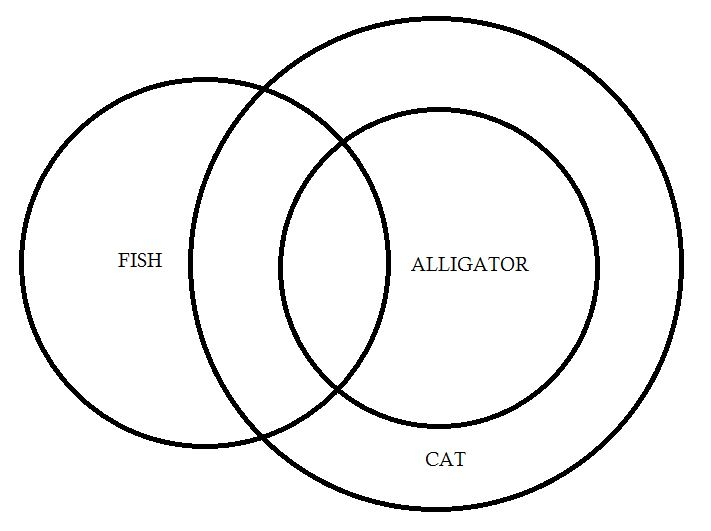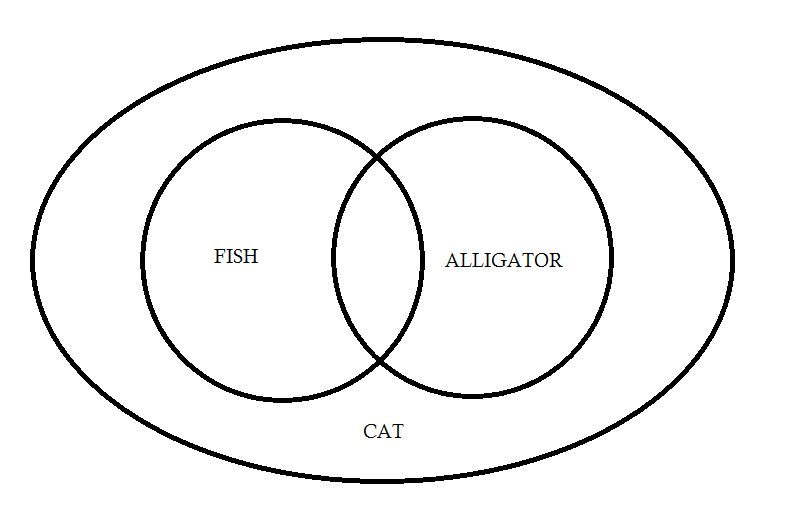Therefore, Only Statement (1) follows. Hence, Option A is correct.

We want circle but subtracting the rectangle
Thus required answer = 21 + 36 + 17 = 74
Hence, option D is the right choice.